/scikit-learn

# sklearn.model_selection.cross_val_score

`sklearn.model_selection.cross_val_score(estimator, X, y=None, groups=None, scoring=None, cv=’warn’, n_jobs=None, verbose=0, fit_params=None, pre_dispatch=‘2*n_jobs’, error_score=’raise-deprecating’)` [source]

Evaluate a score by cross-validation

Read more in the User Guide.

Parameters: `estimator : estimator object implementing ‘fit’` The object to use to fit the data. `X : array-like` The data to fit. Can be for example a list, or an array. `y : array-like, optional, default: None` The target variable to try to predict in the case of supervised learning. `groups : array-like, with shape (n_samples,), optional` Group labels for the samples used while splitting the dataset into train/test set. `scoring : string, callable or None, optional, default: None` A string (see model evaluation documentation) or a scorer callable object / function with signature `scorer(estimator, X, y)`. `cv : int, cross-validation generator or an iterable, optional` Determines the cross-validation splitting strategy. Possible inputs for cv are: None, to use the default 3-fold cross validation, integer, to specify the number of folds in a `(Stratified)KFold`, An object to be used as a cross-validation generator. An iterable yielding train, test splits. For integer/None inputs, if the estimator is a classifier and `y` is either binary or multiclass, `StratifiedKFold` is used. In all other cases, `KFold` is used. Refer User Guide for the various cross-validation strategies that can be used here. Changed in version 0.20: `cv` default value if None will change from 3-fold to 5-fold in v0.22. `n_jobs : int or None, optional (default=None)` The number of CPUs to use to do the computation. `None` means 1 unless in a `joblib.parallel_backend` context. `-1` means using all processors. See Glossary for more details. `verbose : integer, optional` The verbosity level. `fit_params : dict, optional` Parameters to pass to the fit method of the estimator. `pre_dispatch : int, or string, optional` Controls the number of jobs that get dispatched during parallel execution. Reducing this number can be useful to avoid an explosion of memory consumption when more jobs get dispatched than CPUs can process. This parameter can be: None, in which case all the jobs are immediately created and spawned. Use this for lightweight and fast-running jobs, to avoid delays due to on-demand spawning of the jobs An int, giving the exact number of total jobs that are spawned A string, giving an expression as a function of n_jobs, as in ‘2*n_jobs’ `error_score : ‘raise’ | ‘raise-deprecating’ or numeric` Value to assign to the score if an error occurs in estimator fitting. If set to ‘raise’, the error is raised. If set to ‘raise-deprecating’, a FutureWarning is printed before the error is raised. If a numeric value is given, FitFailedWarning is raised. This parameter does not affect the refit step, which will always raise the error. Default is ‘raise-deprecating’ but from version 0.22 it will change to np.nan. `scores : array of float, shape=(len(list(cv)),)` Array of scores of the estimator for each run of the cross validation.

`sklearn.model_selection.cross_validate`
To run cross-validation on multiple metrics and also to return train scores, fit times and score times.
`sklearn.model_selection.cross_val_predict`
Get predictions from each split of cross-validation for diagnostic purposes.
`sklearn.metrics.make_scorer`
Make a scorer from a performance metric or loss function.

#### Examples

```>>> from sklearn import datasets, linear_model
>>> from sklearn.model_selection import cross_val_score
>>> X = diabetes.data[:150]
>>> y = diabetes.target[:150]
>>> lasso = linear_model.Lasso()
>>> print(cross_val_score(lasso, X, y, cv=3))
[0.33150734 0.08022311 0.03531764]
```

## Examples using `sklearn.model_selection.cross_val_score`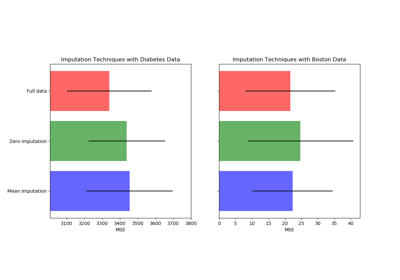Imputing missing values before building an estimator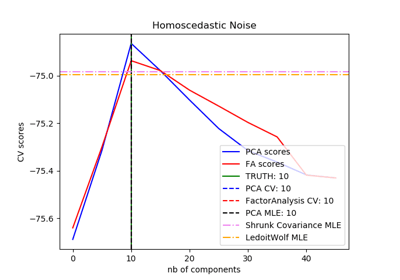Model selection with Probabilistic PCA and Factor Analysis (FA)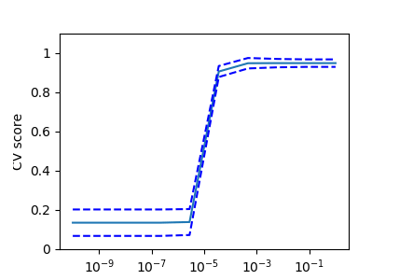Cross-validation on Digits Dataset Exercise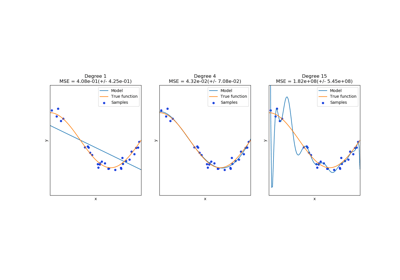Underfitting vs. Overfitting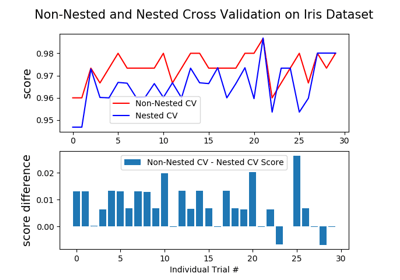Nested versus non-nested cross-validation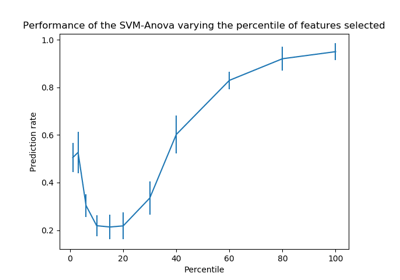SVM-Anova: SVM with univariate feature selection